Home > CC4 > Chapter 11 > Lesson 11.2.6 > Problem11-129

11-129.
1. If f(x) =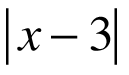+ 5 determine each of the following values. Homework Help ✎

2.  a. f(−1) b.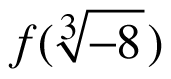c. x if f(x) = 10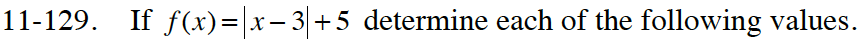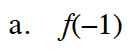Substitute −1 for the
variable x.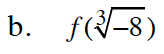$\text{Simplify\,\sqrt{-8}.}$

10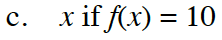One of the answers is x = 8. What is the other?